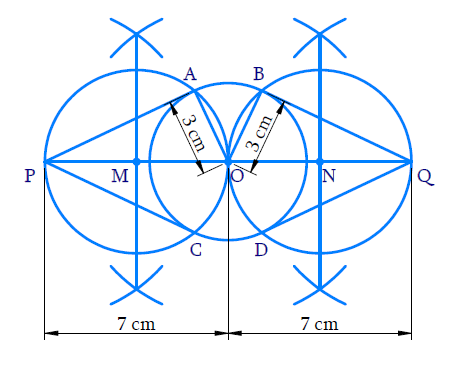# Ex.11.2 Q3 Constructions Solution - NCERT Maths Class 10

Go back to  'Ex.11.2'

## Question

Draw a circle of radius $$3 \,\rm{cm}$$. Take two points $$P$$ and $$Q$$ on one of its extended diameter each at a distance of $$7 \,\rm{cm}$$ from its center. Draw tangents to the circle from these two points $$P$$ and $$Q$$.

Video Solution
Constructions
Ex 11.2 | Question 3

## Text Solution

#### Steps:Steps of construction:

(i) Draw a circle with $$O$$ as centre and radius is $$3 \,\rm{cm}$$.

(ii) Draw a diameter of it extend both the sides and take points $$P$$, $$Q$$ on the diameter such that \begin{align}\rm{OP}=\rm{OQ}=7 \rm{cm}\end{align}

(iii) Draw the perpendicular bisectors of $$OP$$ and $$OQ$$ to intersect $$OP$$ and $$OQ$$ at $$M$$ and $$N$$ respectively.

(iv) With $$M$$ as centre and $$OM$$ as radius draw a circle to cut the given circle at $$A$$ and $$C$$. With $$N$$ as centre and $$ON$$ as radius draw a circle to cut the given circle at $$B$$ and $$D$$.

(v) Join $$PA$$, $$PC$$ $$QB$$, $$QD$$

$$PA$$,$$PC$$ and $$QB$$,$$QD$$ are the required tangents from $$P$$ and $$Q$$ respectively.

Proof:

$$\angle {{PAO}} =\angle {{QBO}}=90^\circ$$ (Angle in a semi - circle)

$$\therefore {PA} \perp {AO},\; {QB} \perp {BO}$$

Since $$OA$$ and $$OB$$ are the radii of the given circle, $$PA$$ and QB are its tangents at $$A$$ and $$B$$ respectively.

In right angle triangle $$PAO$$ and $$QBO$$

$$OP = OQ = 7 \,\rm{cm }$$ (By construction)

$$OA = OB = 3\,\rm{cm}$$ (radius of the given circle)

\begin{align} {{P}}{{{A}}^{{2}}}&={{ (OP}}{{{)}}^{{2}}}{{ -(OA}}{{{)}}^{{2}}}\,\,\,\,\,\,\,\\ &= {{ (7}}{{{)}}^{{2}}}{{ - (3}}{{{)}}^{{2}}}\\ &= {{ 49 - 9}}\\ &={{ 40}}\\ {{PA }}&=\sqrt {{{40}}} \\ &={{ 6}}{{.3}}\,\,{\rm{(approx)}} \end{align}

And

\begin{align} {{Q}}{{{B}}^{{2}}}&={{(OQ}}{{{)}}^{{2}}}{{ - (OB}}{{{)}}^{{2}}}\,\,\,\,\,\,\,\\ &={{(7}}{{{)}}^{{2}}}{{ - (3}}{{{)}}^{{2}}}\\ &={{ 49 - 9}}\\ &={{ 40}}\\ {{QB }}&=\sqrt {{{40}}} \\ &={{ 6}}{{.3}}\,\,{\rm{(approx)}} \end{align}

Learn from the best math teachers and top your exams

• Live one on one classroom and doubt clearing
• Practice worksheets in and after class for conceptual clarity
• Personalized curriculum to keep up with school